# Resistivity calculations using the MD-Landauer method¶

Version: 2017.0

The bulk resistivity is an intrinsic property quantifying how strongly a material disrupts a flux of electronic current. In pristine metals, the main cause of the resistivity at room temperature is electron-phonon interaction, i.e., electron scattering due to the interaction with the vibrations of the lattice. The higher the temperature, the larger becomes the vibrational amplitude of the lattice and hence the scattering probability, leading to linear increase of the resisitivity to the temperature.

This case study aims at introducing the MD-Landauer method, which is a conceptually simple methodology for calculating the temperature-dependent resistivity based on combined molecular-dynamics (MD) and electronic-transport calculations within the framework of the Landauer formalism (check the tutorial Transport calculations with QuantumATK). Despite its simplicity, the MD-Landauer method has several advantages over other more rigorous methods to calculate bulk resistivities (see, e.g., Refs. [cBMOrdejon+02] and [cSPS+10], and also the tutorial Phonon-limited mobility in graphene using the Boltzmann transport equation), as it takes naturally into account the anharmonic effects in describing lattice vibrations, and is applicable to non-crystalline systems as well.

The case study consists of three sections, through which we reproduce some results for bulk gold recently reported in the paper “Electron-phonon scattering from Green’s function transport combined with Molecular Dynamics: Applications to mobility predictions” [cMPS+17]. Section 1. Theory and numerical procedure provides some theoretical and methodological details. Section 2. Calculation setup then describes how to setup an MD-Landauer calculation in QuantumATK. Finally, Sec. 3. Data analysis is devoted to explaining how to analyze the data files and compute the resistivity.

Note

The numerical parameters employed in the calculations below have been tuned to achieve a compromise between computational efficiency and accuracy. The results will hence slightly differ from those presented in Ref. [cMPS+17].

Note

QuantumWise case studies are primarily directed at experienced users of QuantumATK. Instructions are deliberately concise in order to focus mostly on the science.

For more basic details on how to use QuantumATK, please refer to the tutorials Introduction to QuantumATK and Transport calculations with QuantumATK.## 1. Theory and numerical procedure¶

The figure above displays an illustrative example of a device configuration modeling bulk gold, for which the MD-Landauer calculations are performed. There are two semi-infinite electrodes and, in between, a central region which, importantly, consists of three parts: the MD region with length $${\mathcal L}$$ and the electrode copy on its either side. An incoming flux of electrons from one of the electrodes interacts with phonons in the MD region, and is transmitted with a certain probability to the other electrode. Assuming that the electrodes and their replicas always remain equilibrium at temperature $$T$$, MD calculations are performed by allowing the atoms to move only in the MD region for a few nanoseconds, starting with random initial velocities based on the Maxwell-Boltzmann distribution. At the end of each MD calculations, the transmission coefficient is computed as

$\mathscr{T}[E;{\bf x}(T),\mathcal{L}] = {\rm Tr} \big\{ G^{\rm r}[E;{\bf x}(T),\mathcal{L}] \Gamma_{\rm L}(E) G^{\rm a}[E;{\bf x}(T),\mathcal{L}] \Gamma_{\rm R}(E) \big\},$

where $$G^\rm {r/a}[E;{\bf x}({\it T}),\mathcal{L}]$$ represents the retarded/advanced Green’s function, and $$\Gamma_{\rm L/R}(E)$$ is the width in the left/right electrode (see, e.g., Ref. [cDat97] for details of the derivation). Note that the Green’s functions and thus the transmission coefficient are both functions of $$E$$ and parametrically depend on the set of displacement coordinates of atoms $${\bf x}({\it T})$$ as well as $$\mathcal{L}$$. The Landauer formula then provides the conductance as a function of $$\mathcal{L}$$ and $$T$$:

$\mathscr{G}(\mathcal{L},T) = \frac{2e^2}{h} \int \langle \mathscr{T}[E;{\bf x}(T),\mathcal{L}] \rangle \left[ -\frac{\partial n_{\rm F}(E,\epsilon_{\rm F},T)}{\partial E} \right]dE,$

where $$n_{\rm F}(E,\epsilon_{\rm F},T)$$ represents the Fermi-Dirac distribution function with $$\epsilon_{\rm F}$$ being the Fermi energy, and $$\langle\cdots\rangle$$ means taking average over a set of MD calculations. The resistance is defined as the inverse of the conductance, i.e.,

$\begin{split}1/\mathscr{G}(\mathcal{L},T) &=& R(\mathcal{L},T) \nonumber \\ &\equiv& R_{\rm c}+\rho_{\rm 1D}(T)\mathcal{L},\end{split}$

where the second line defines the one-dimensional (1D) resistivity $$\rho_{\rm 1D}(T)$$. The bulk resitivity is then given by

$\rho_{\rm bulk}(T)=\rho_{\rm 1D}(T)\cdot A,$

where $$A$$ is the cross section of the device normal to the transport direction.

Dividing the procedure into three steps as follows will help clarify how to compute the 1D and bulk resistivies:

• Calculation of the lattice geometries:
For a given value of $$T$$ and a set of values of $$\mathcal{L}$$, several MD calculations with random initial velocities are performed. For each calculation, the last snapshot of the MD run is taken.
• Calculation of the resistance:
For each set of snapshots with a given length $$\mathcal{L}$$, the averaged transmission coefficient is computed, which is then used to calculate the conductance $$\mathscr{G}(\mathcal{L},T)$$ and the resistance $$R(\mathcal{L},T)$$.
• Calculation of the finite-temperature resistivity:
The 1D and bulk resistivities at the temperature $$T$$ are calculated.

The rest of this case study details each step as well as the procedure to construct a device configuration of bulk gold.

## 2. Calculation setup¶

### 2.1. Construction of the DeviceConfiguration¶

Tip

This subsection presents a detailed how-to guidline for preparing a device configuation of bulk gold, and finally provides an QuantumATK Python script, Au100_L3.py. To save time, one may just download the script and use it.

In the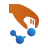Builder, go to Add ‣ From Database… and select “Gold”:

Then use the Builders ‣ Surface (Cleave) tool as follows to create a 3x3 Au bulk supercell with a thickness of 10 layers:

1. Create a  surface by setting h=0 and k=l=0.
2. Define the surface lattice vectors by $${\bf v}_1=3{\bf u}_1$$ and $${\bf v}_2=3{\bf u}_2$$.
3. Select “Periodic and normal (electrode)” and set the thickness to 10 layers.

Finally, use the Device Tools ‣ Device from Bulk plugin to create a DeviceConfiguration. Set the length of both right and left electrodes to be the minimum value 4.07825 Å.

Save the device configuration as “Au100_L3.py,” where “L3” indicates $$\mathcal{L}=3$$ in terms of the number of electrode repetitions. Following a similar procedure, one will be able to easily construct the device configurations for $$\mathcal{L}=4$$ and $$5$$, and save them with proper file names.

### 2.2. Setup of the script¶

Send the device configuration toScripter by clicking, and add four blocks in the order as follows:

In the section Global IO options, change the name of the output file to “Au100_L3_T300.nc.” The parameters in each block should then be specified as follow.

#### MD section¶

The set of two blocks,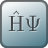and, in the section (1) highlighted in yellow, is meant to account for the MD calculations.

Open theNew Calculator block, then select ATK-ForceField from the available Calculators, and set “EAM_Au_Sheng_2011[cSKC+11] in Parameter set.

Note

For QuantumATK-versions older than 2017, the ATK-ForceField calculator can be found under the name ATK-Classical.

In theMolecularDynamics block, choose the Langevin thermostat [cGRdV+12] and set the rest of the MD parameters as shown below.

Important

In order to describe a random distribution of initial velocities in the MD simulations, remember to set the initial velocity type to Maxwell-Boltzmann.

#### Landauer calculation section¶

The set of two blocks,and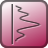, in the section (2) highlighted in yellow is meant to account for the DFTB-based transport calculations based on the Landauer approach.

Click on theNew Calculator block. Select “ATK-SE Slater_Koster (Device)” from the list of available Calculators and set up the parameters as shown below.

Important

Remember to untick “No SCF iteration” to carry out the calculations self-consistently.

Click on theTransmissionSpectrum object, and set the parameters as shown below.

Note

The choice of the “Krylov” method is of particular importance as it accelerates the convergence in the SCF calculations.

### 2.3. Edit the python script¶

Making fully use of GUI supported by QuantumATK, the device configuration has been so far constructed with the necessary calculators and analyzer attached. The MD-Landauer method for computing the conductance, resistance, and resistivity, however, requires performing the calculation, for each of given values of $$\mathcal{L}$$ and $$T$$, several times to obtain averaged transmission coefficient $$\langle\mathscr{T}[E;{\bf x}(T),\mathcal{L}]\rangle$$. To this end, the python script needs to be slightly modified by hand.

By clicking theicon, send the script to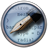Editor. Highlight the whole script and press the Tab key on your keyboard to indent every line. Then edit the script as shown below, and save it as “Au100_L3_T300.py” To make sure that there is no mistake in your code, download Au100_L3_T300.py and compare the two scripts.

  1 2 3 4 5 6 7 8 9 10 11 12 13 14 15 16 17 # -*- coding: utf-8 -*- for run in range(0,10): # ------------------------------------------------------------- # Two-probe Configuration # ------------------------------------------------------------- # ------------------------------------------------------------- # Left Electrode # ------------------------------------------------------------- # Set up lattice vector_a = [8.65127469112, 0.0, 0.0]*Angstrom vector_b = [0.0, 8.65127469112, 0.0]*Angstrom vector_c = [0.0, 0.0, 4.07825]*Angstrom left_electrode_lattice = UnitCell(vector_a, vector_b, vector_c) 

Note

Since the MD and Landauer sections are all placed under a loop, this script evaluates the transmission coefficient 10 times starting with different values of initial velocity, and saves each result with a unique ID number (gIDxxx) attached.

Note

Following exactly the same procedure except employing different values of $$\mathcal{L}$$, e.g., $$\mathcal{L}=$$ 4 and 5, one can easily prepare the scripts for systematic implementation of the MD-Landauer calculations at $$T$$ =300 K. To save time, one may download Au100_L4_T300.py and Au100_L5_T300.py, and use them.

## 3. Data analysis¶

Click theicon, send the script toJob Manager, and run it. The calculation takes 5-6 hours on 8 cores. The set of transmission coefficients should then be averaged for the analysis. This section hence focuses on how to analyze the results and obtain the 1D and bulk resistivities.

### 3.1. Transmission functions, conductance, and resistance¶

QuantumATK provides a useful plugin to make comparison of the transmission functions. Select all the transmission functions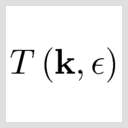in LabFloor and click the Compare Data... button in Plugin Bar.

A window will pup up and display all the transmission functions $$\big\{\mathscr{T}[E;{\bf x}(T),\mathcal{L}]\big\}_{{\bf x}(T)}$$ for $$T=300$$ K and $$\mathcal{L}=3$$.

To compute the average of them, download the script analysis.py and run it as atkpython analysis.py in the same directory of the nc. The analysis will finish in a few seconds, and provide a list of information about the length of the MD-region, and the averaged conductance and resistance:

There also appears a pup-up window, in which black lines show the transmission coefficients as before, and a thick red line represents their average $$\langle\mathscr{T}[E;{\bf x}(T),\mathcal{L}]\rangle$$.

### 3.2. Resistivity¶

To compute the resistance at $$T=300$$ K also for $$\mathcal{L}=$$ 0, 4, and 5, download the scripts Au100_L0_T0.py, Au100_L4_T300.py, and Au100_L5_T300.py. Run them as atkpython Au100_Lx_Txxx.py, and analyze the results. One will then obtain a list of resistances, indicating the linear dependence of the resistance on $$\mathcal{L}$$:

Length of the MD-region, $$\mathcal{L}$$
Resistance
(# of electrode repetitions) (Å) (Ω)
0 0 1482.9
3 12.235 1535.1
4 16.313 1555.2
5 20.391 1571.9

Note

The resistance for $$\mathcal{L}=0$$ is computed using the script Au100_L0_T0.py, which implements no MD calculation, and because of it, is hence named with “T0.” This script aims at evaluating the contact resistance.

Let us then compute the 1D and bulk resistivities, which requires the value of the cross section. Noting the primitive vectors defined in lines 14 and 15 of “Au100_L3_T300.py,” i.e.,

 14 15  vector_a = [8.65127469112, 0.0, 0.0]*Angstrom vector_b = [0.0, 8.65127469112, 0.0]*Angstrom 

the cross section, in this case, is given by $$A=$$ $$\|\hspace{-1mm}$$ vector_a $$\|\hspace{-1mm}$$ × $$\|\hspace{-1mm}$$ vector_b $$\|\hspace{-1mm}$$ = 74.84 Å $$^2$$. Using this value, and also the values of $$\mathcal{L}$$ and resistance listed in the table above, one can evaluate the 1D and bulk resistivities at $$T=300$$ K. To this end, download resistivity.py, and, after entering the values in the table into the INPUT SECTION of the script, run it as atkpython resistivity.py.

  6 7 8 9 10 11 12 #--------------------------------------------------------------------# # INPUT SECTION | #--------------------------------------------------------------------# cs_area = 8.65127469112**2 lengths_list = [0,12.235,16.313,20.391] resistances_list = [1482.9,1535.1,1555.2,1571.90] output_filename = 'resistivity.png' 

The script interpolates the data points by means of the least squares method and provides the values of the contact resistance, as well as the 1D and bulk resistivities at $$T=300$$ K:

A red diamond in the figure below is the bulk resistivity at $$T=300$$ K, and is compared with purple points representing the results of the MD-Landauer calculations shown in Fig. 7(b) of Ref. [cLid97]. Because of the reduced numerical condition employed in this case study, there exists a small difference. The figure also displays black squares representing the experimental result [cLid97], and proves the qualitative agreement with the MD-Landauer result.